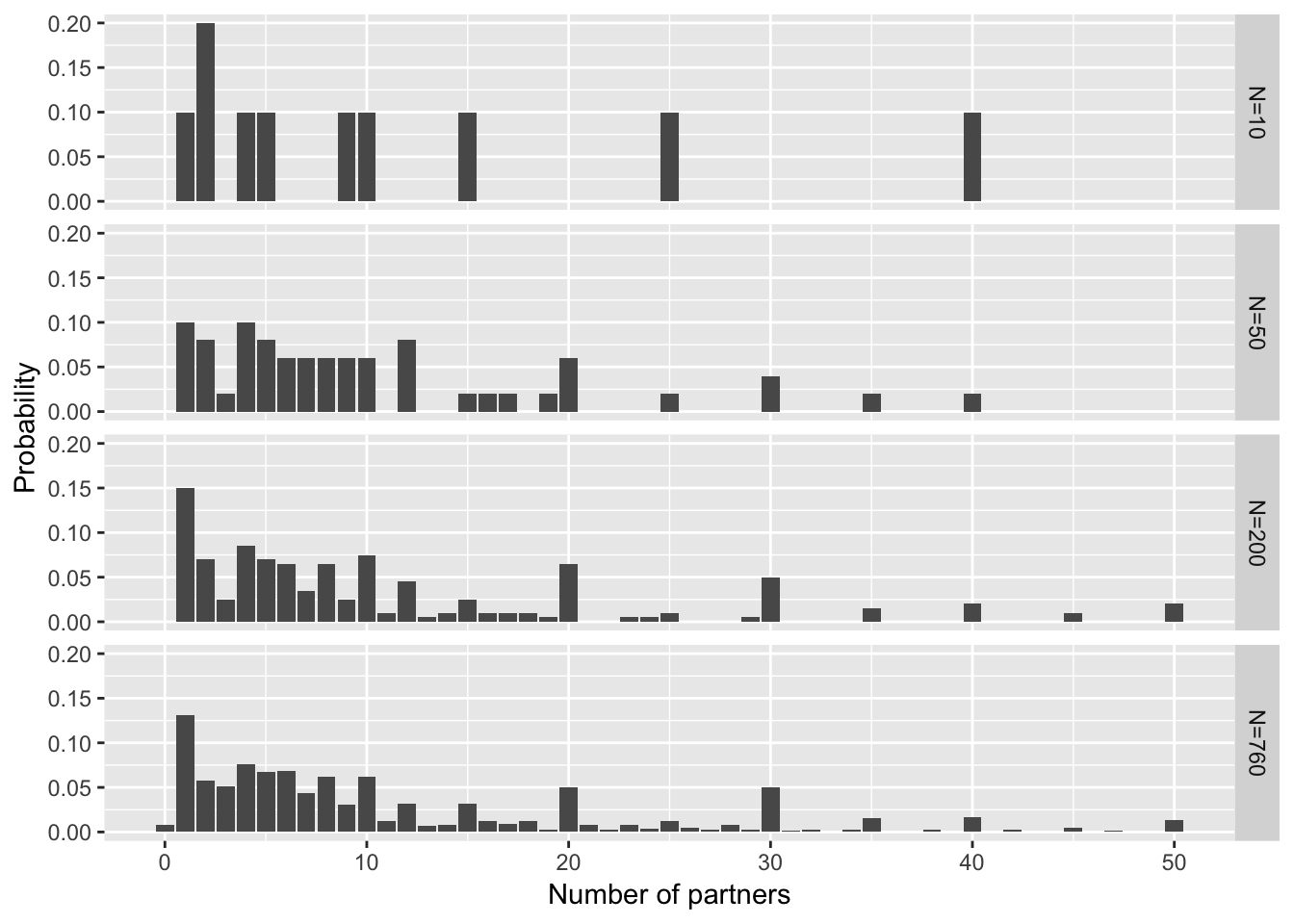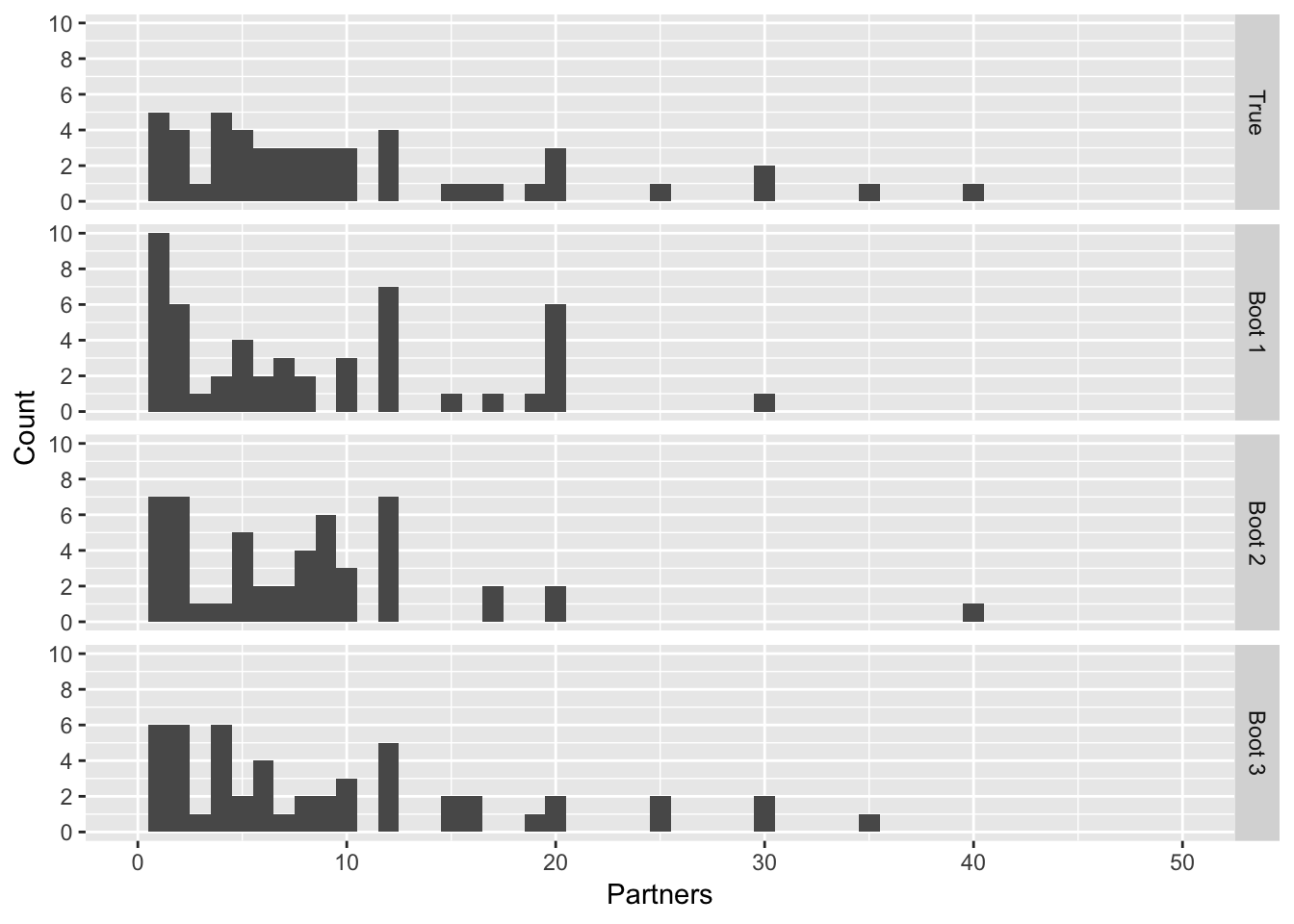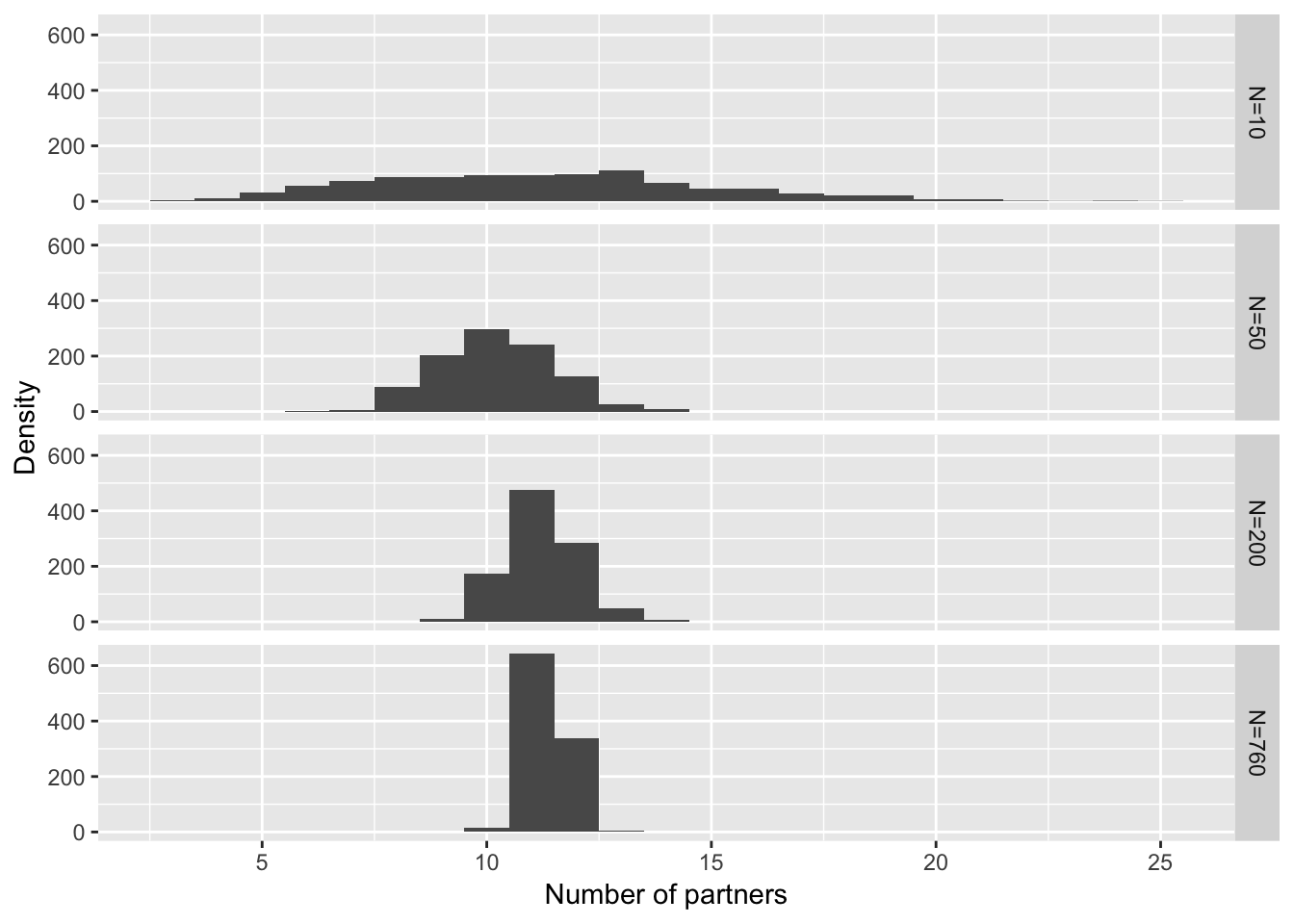Data are contained in 02-4-sexual-partners-counts-x.csv, and comprise the distribution of the reported lifetime number of opposite-sex partners for men and women aged 35-44. These are then truncated at a maximum of 50, for illustrative purposes.

The data is stored at interim stages, for example, “# Book figure 7-1 datasnatch here” for the exact source-points of the CSV’s used. Code for the book figures is at the bottom.

#Data Preparation

# libraries
library("ggplot2")
library("reshape2")

# set seed for reproducibility  - subsequent results are very sensitive to this seed!!
# set.seed(12321)
set.seed(22222)

### Output Params

LabelPts <- 14
MainPts <- 15
AxisPts <- 14
DefaultTheme <- theme(strip.text=element_text(size=LabelPts),
plot.title=element_text(size=MainPts),
axis.title=element_text(size=AxisPts))

#   - percentage of respondents with given lifetime number of sexual parteners
SexData50 <- SexData[SexData$NumPartners<51,] attach(SexData50) ### Sample size NMen <- sum(MenCount) Men.data=rep(NumPartners,MenCount) # Renormalise percentages to allow for truncation to 50 PMen <- MenPercent / sum(MenPercent) ### Sampling parameters sample_n <- function(n, prob=PMen){ sample(NumPartners, n, prob=prob, replace=TRUE) } # sample n with replacement from data distribution ### Single samples SubSampleSizes <- c(10, 50, 200, NMen) #draw nested subsamples Samples4Boot <- lapply(1:4, function(i){rnorm(10)}) Samples4Boot[] <- Men.data # this is actual distribution and not a sample for(i in 1:3){ Size_ <- SubSampleSizes[4-i] Sample_ <- Samples4Boot[[5-i]] Samples4Boot[[4-i]] <- sample(Sample_, Size_, replace=F) # should not be with replacement } SamplesMeans <- sapply(Samples4Boot, mean) SamplesMedians <- sapply(Samples4Boot, median) SamplesMeans ##  8.30 10.52 12.20 11.35 SamplesMedians ##  9.0 7.5 8.0 7.0 ### 7-1 Overlaid frequency plots for different sample sizes SamplesKeys <- sapply(Samples4Boot , function(s){as.integer( dimnames( table( s ) )[] )} ) SamplesCounts <- sapply(Samples4Boot , function(s){ x <- as.integer( table( s )) x/sum(x) }) FreqDF <- cbind(melt(SamplesCounts, id="x")[,1], melt(SamplesKeys, id="x")) names(FreqDF) <- c("count", "partners", "size") FreqDF=cbind(FreqDF,ordering=FreqDF$size)
for(i in 1:4){
FreqDF$size[FreqDF$size == i] = SubSampleSizes[i]
}
FreqDFSizes <- as.factor(FreqDF$size) levels(FreqDFSizes) <- sapply(SubSampleSizes, function(n){paste("N=",n,sep="")}) FreqDF$size <- FreqDFSizes

# Book figure 7-1 datasnatch here
# write.csv(FreqDF, "7-1 datasnatch.csv") # WARNING: uncommenting will overwrite existing file and lose alterations to appropriately label and reorder

# 7-2 Bootstrap resamples from N=50 subsample

SubSamp50 <- Samples4Boot[]
NumResamps <- 4 # 3+1

BootResamples50 <- lapply(1:NumResamps, function(i, sub_samp=SubSamp50){sample(sub_samp, size=length(sub_samp), replace=TRUE)})
BootResamples50[] <- SubSamp50 #overwrite with the original

# get means [done very clumsily]
resamplemeans=rep(0,4)
for(i in 1:NumResamps){
resamplemeans[i]=mean(BootResamples50[[i]])
}
resamplemeans
##  10.52  8.38  9.72  9.78
samples=unlist(lapply(1:4, function(n){rep(n, length(SubSamp50))}))
sampleid <- as.factor(samples)
levels(sampleid) <- c("True", "Boot 1", "Boot 2", "Boot 3")
BootFrame50 <- data.frame(sampleid=sampleid,
partners=unlist(BootResamples50), ordering=samples )

# Book figure 7-2 datasnatch here
#write.csv(BootFrame50, "7-2 datasnatch.csv") # WARNING: uncommenting will overwrite existing file and lose alterations to appropriately label and reorder

### 7-3 Bootstrap on subsample

NumSubSamples <- 1000

BootSampleMenMeans <- lapply(Samples4Boot, function(sub_samp){
sapply(1:NumSubSamples, function(i){mean( sample(sub_samp, size=length(sub_samp), replace=TRUE))} )
})
BootSampleMenMedians <- lapply(Samples4Boot, function(sub_samp){
sapply(1:NumSubSamples, function(i){median( sample(sub_samp, size=length(sub_samp), replace=TRUE))} )
})

# get 95% intervals [done very clumsily]
resample_low=resample_high=rep(0,4)
for(i in 1:4){
resample_low[i]=quantile(BootSampleMenMeans[[i]],0.025)
resample_high[i]=quantile(BootSampleMenMeans[[i]],0.975)
}
resample_low
##   5.30000  7.73950 10.54425 10.54855
resample_high
##  11.50000 13.82050 13.81537 12.20276
# Set up for stacked histograms
sizes=unlist(lapply(SubSampleSizes, function(n){rep(n, NumSubSamples)}))
Sizes4BootFrame <- as.factor(sizes)
levels(Sizes4BootFrame) <- sapply(SubSampleSizes, function(n){paste("N=",n,sep="")})
BootFrame <- data.frame(size=Sizes4BootFrame,
means=unlist(BootSampleMenMeans),
medians=unlist(BootSampleMenMedians), ordering=sizes)

# Book figure 7-3 datasnatch here
#write.csv(BootFrame, "7-3 datasnatch.csv") # WARNING: uncommenting will overwrite existing file and lose alterations to appropriately label and reorder

Figure 7-1

library(ggplot2)
p <- ggplot(aes(x=partners, y=count), data=ClippedDF) # assign plot from dataset to p
p <- p + geom_bar(stat="identity") # assign bar-chart type to plot
p <- p + facet_grid(reorder(size,ordering) ~ .) # assign facet grid on size, with order defined by data column 'ordering'
p <- p + xlab("Number of partners")+ ylab("Probability") # assigns the axis labels
p # displays plotFigure 7.1 The bottom panel shows the distribution of responses of all 760 men in the survey with partner count up to 50. Individuals are successively sampled at random from this group, pausing at samples of size 10, 50, 200, producing the distributions in the top three panels. Smaller sample sizes show a more variable pattern, but the shape of the distribution gradually approaches that of the whole group of 760 men. Values above 50 partners are not shown.

Figure 7-2

BootFrame50 <- read.csv("7-2 datasnatch.csv") # assign data to dataframe, BootFrame50
p <- ggplot(BootFrame50) # assign dataframe to plot, p
p <- p + geom_histogram(aes(x=partners), binwidth=1) # assign histogram chart-type to p
p <- p + facet_grid(reorder(sampleid,ordering) ~ .) # assign facet grid on size, with order defined by data column 'ordering'
p <- p + scale_x_continuous(name="Partners", limits=c(0, 50))
p <- p + scale_y_continuous(name="Count", limits=c(0, 10), breaks=seq(0,10,2))
p
## Warning: Removed 8 rows containing missing values (geom_bar).Figure 7.2 The original sample of 50 observations, and three ‘bootstrap’ r es amples, each based on sampling 50 observations at random from the original set, replacing the sampled data-point each time. For example, an observation of 30 partners occurs twice in the original sample. These data-points were sampled once in the first bootstrap sample, not at all in the second, and twice in the third.

Figure 7-3 Plot the bootstrap means

BootFrame <- read.csv("7-3 datasnatch.csv") # assign data to dataframe, BootFrame
p <- ggplot(aes(x=means), data=BootFrame) # assign plot object defined by dataframe to p
p <- p + geom_histogram(binwidth=1) # assign histogram chart-type to the plot
p <- p + facet_grid(reorder(size, ordering) ~ .) # define facet by size on plot, with reordering defined by data column 'ordering'
p <- p + xlab("Number of partners") + ylab("Density") # assign axis labels
p # draws the plotFigure 7.3 Distribution of sample means of 1,000 bootstrap resamples, for each of the original samples shown in Figure 7.1. The variability of the sample means of the bootstrap resamples decreases as the sample size increases.